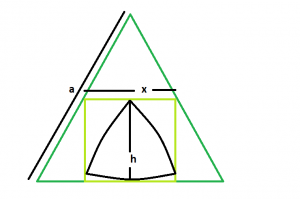# Biggest Reuleaux Triangle inscribed within a Square inscribed in an equilateral triangle

Given here is an equilateral triangle of sidelength a which inscribes a square which in turn inscribes a reuleaux triangle. The task is to find the maximum possible area of this reuleaux triangle.

Examples:

```Input : a = 5
Output : 3.79335

Input : a = 9
Output : 12.2905
```

## Recommended: Please try your approach on {IDE} first, before moving on to the solution.Approach: We know that the side of the square inscribed within an equilateral triangle of side lengthis, x = 0.464*a (Please refer here).

Also, in the reuleaux triangle, h = x.

```A = 0.70477*h2
= 0.70477*(0.464*a)2
```

Below is the implementation of the above approach:

## C++

 `// C++ Program to find the biggest Reuleaux triangle ` `// inscribed within in a square which in turn ` `// is inscribed within an equilateral triangle ` `#include ` `using` `namespace` `std; ` ` `  `// Function to find the biggest reuleaux triangle ` `float` `Area(``float` `a) ` `{ ` ` `  `    ``// side cannot be negative ` `    ``if` `(a < 0) ` `        ``return` `-1; ` ` `  `    ``// height of the reuleaux triangle ` `    ``float` `x = 0.464 * a; ` ` `  `    ``// area of the reuleaux triangle ` `    ``float` `A = 0.70477 * ``pow``(x, 2); ` ` `  `    ``return` `A; ` `} ` ` `  `// Driver code ` `int` `main() ` `{ ` `    ``float` `a = 5; ` `    ``cout << Area(a) << endl; ` ` `  `    ``return` `0; ` `} `

## Java

 `// Java Program to find the biggest Reuleaux triangle ` `// inscribed within in a square which in turn ` `// is inscribed within an equilateral triangle ` ` `  `class` `GFG ` `{ ` `     `  `// Function to find the biggest reuleaux triangle ` `static` `float` `Area(``float` `a) ` `{ ` ` `  `    ``// side cannot be negative ` `    ``if` `(a < ``0``) ` `        ``return` `-``1``; ` ` `  `    ``// height of the reuleaux triangle ` `    ``float` `x = ``0``.464f * a; ` ` `  `    ``// area of the reuleaux triangle ` `    ``float` `A = ``0``.70477f * (``float``)Math.pow(x, ``2``); ` ` `  `    ``return` `A; ` `} ` ` `  `// Driver code ` `public` `static` `void` `main (String[] args) ` `{ ` `    ``float` `a = ``5``; ` `    ``System.out.println(String.format(``"%.5f"``, Area(a))); ` `} ` `} ` ` `  `// This code is contributed by chandan_jnu `

## Python3

 `# Python3 Program to find the biggest  ` `# Reuleaux triangle inscribed within ` `# in a square which in turn is inscribed ` `# within an equilateral triangle ` `import` `math as mt ` ` `  `# Function to find the biggest ` `# reuleaux triangle ` `def` `Area(a): ` ` `  `    ``# side cannot be negative ` `    ``if` `(a < ``0``): ` `        ``return` `-``1` ` `  `    ``# height of the reuleaux triangle ` `    ``x ``=` `0.464` `*` `a ` ` `  `    ``# area of the reuleaux triangle ` `    ``A ``=` `0.70477` `*` `pow``(x, ``2``) ` ` `  `    ``return` `A ` ` `  `# Driver code ` `a ``=` `5` `print``(Area(a)) ` ` `  `# This code is contributed by  ` `# Mohit Kumar 29 `

## C#

 `// C# Program to find the biggest Reuleaux  ` `// triangle inscribed within in a square  ` `// which in turn is inscribed within an  ` `// equilateral triangle ` `using` `System; ` ` `  `class` `GFG ` `{ ` `     `  `// Function to find the biggest  ` `// reuleaux triangle ` `static` `float` `Area(``float` `a) ` `{ ` ` `  `    ``// side cannot be negative ` `    ``if` `(a < 0) ` `        ``return` `-1; ` ` `  `    ``// height of the reuleaux triangle ` `    ``float` `x = 0.464f * a; ` ` `  `    ``// area of the reuleaux triangle ` `    ``float` `A = 0.70477f * (``float``)Math.Pow(x, 2); ` ` `  `    ``return` `A; ` `} ` ` `  `// Driver code ` `public` `static` `void` `Main () ` `{ ` `    ``float` `a = 5; ` `    ``Console.WriteLine(String.Format(``"{0,0:#.00000}"``,  ` `                                          ``Area(a))); ` `} ` `} ` ` `  `// This code is contributed by Akanksha Rai `

## PHP

 `

Output:

```3.79335
```

Attention reader! Don’t stop learning now. Get hold of all the important DSA concepts with the DSA Self Paced Course at a student-friendly price and become industry ready.

My Personal Notes arrow_drop_upCheck out this Author's contributed articles.

If you like GeeksforGeeks and would like to contribute, you can also write an article using contribute.geeksforgeeks.org or mail your article to contribute@geeksforgeeks.org. See your article appearing on the GeeksforGeeks main page and help other Geeks.

Please Improve this article if you find anything incorrect by clicking on the "Improve Article" button below.